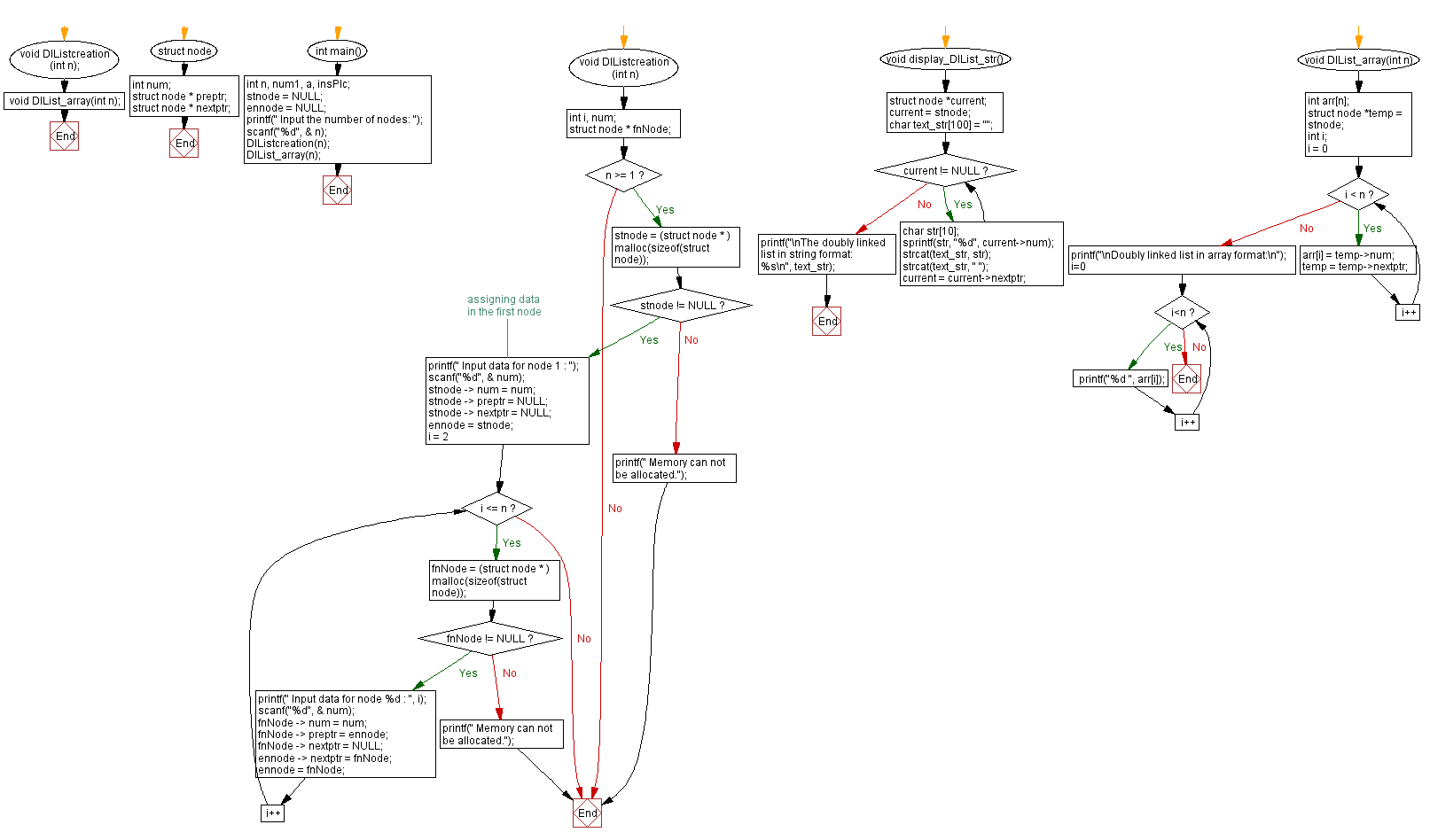﻿ C Program: Convert a Doubly Linked list into a array

# C Exercises: Convert a Doubly Linked list into a array

## C Doubly Linked List : Exercise-22 with Solution

Write a C program to convert a doubly linked list into an array and return it.

Sample Solution:

C Code:

``````#include<stdio.h>
#include <stdlib.h>
#include <string.h>

struct node {
int num;
struct node * preptr;
struct node * nextptr;
}* stnode, * ennode;

void DlListcreation(int n);
void DlList_array(int n);

int main() {
int n, num1, a, insPlc;
stnode = NULL;
ennode = NULL;
printf(" Input the number of nodes: ");
scanf("%d", & n);
DlListcreation(n);
DlList_array(n);
}

void DlListcreation(int n) {
int i, num;
struct node * fnNode;

if (n >= 1) {
stnode = (struct node * ) malloc(sizeof(struct node));
if (stnode != NULL) {
printf(" Input data for node 1 : "); // assigning data in the first node
scanf("%d", & num);
stnode -> num = num;
stnode -> preptr = NULL;
stnode -> nextptr = NULL;
ennode = stnode;
for (i = 2; i <= n; i++) {
fnNode = (struct node * ) malloc(sizeof(struct node));
if (fnNode != NULL) {
printf(" Input data for node %d : ", i);
scanf("%d", & num);
fnNode -> num = num;
fnNode -> preptr = ennode;
fnNode -> nextptr = NULL;
ennode -> nextptr = fnNode;
ennode = fnNode;
} else {
printf(" Memory can not be allocated.");
break;
}
}
} else {
printf(" Memory can not be allocated.");
}
}
}

void display_DlList_str() {
struct node *current;
current = stnode;
char text_str = "";
while (current != NULL) {
char str;
sprintf(str, "%d", current->num);
strcat(text_str, str);
strcat(text_str, " ");
current = current->nextptr;
}
printf("\nThe doubly linked list in string format: %s\n", text_str);
}
void DlList_array(int n) {
int arr[n];
struct node *temp = stnode;
int i;
for (i = 0; i < n; i++) {
arr[i] = temp->num;
temp = temp->nextptr;
}
printf("\nDoubly linked list in array format:\n");
for (i=0; i<n; i++)
printf("%d ", arr[i]);
}
```
```

Sample Output:

``` Input the number of nodes: 4
Input data for node 1 : 10
Input data for node 2 : 11
Input data for node 3 : 12
Input data for node 4 : 13

Doubly linked list in array format:
10 11 12 13
```

Flowchart :C Programming Code Editor:

What is the difficulty level of this exercise?

﻿